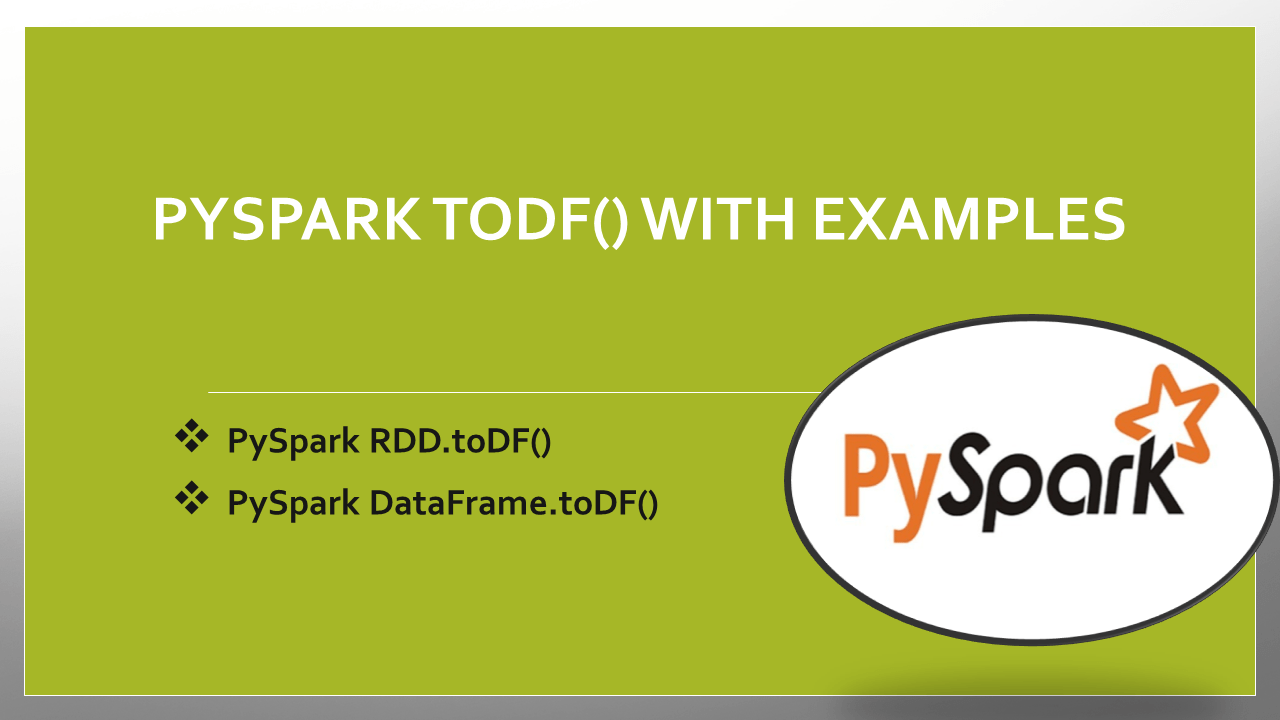# PySpark toDF() with Examples

The pyspark.sql.DataFrame.toDF() function is used to create the DataFrame with the specified column names it create DataFrame from RDD. Since RDD is schema-less without column names and data type, converting from RDD to DataFrame gives you default column names as `_1``_2` and so on and data type as String. Use DataFrame printSchema() to print the schema to console.

Key Points of PySpark toDF()

• toDF() Returns a DataFrame
• The toDF() is present on both RDD and DataFrame data structures.
• The toDF(), by default, crates the column name as _1 and _2.
• toDF() also supports taking column names as a list or Schema as an argument.

## 1. PySpark RDD.toDF()

PySpark RDD `toDF()` has a signature that takes arguments to define column names of DataFrame as shown below. This function is used to set column names when your DataFrame contains the default names or change the column names of the entire Dataframe.

### 1.1 Syntax toDF()

Following is the Syntax of RDD.toDF()

``````
# Syntax
toDF()
toDF(cols)
toDF(schema)
toDF(schema, sampleRatio)
``````

### 1.2 toDF() Example

PySpark RDD contains different syntaxes of the toDF() function. Default function that doesn’t take the column names or Scheme returns a DataFrame with columns names _1, _2 _3 e.t.c

``````
# Imports
from pyspark.sql import SparkSession

# Create SparkSession
spark = SparkSession.builder \
.appName('SparkByExamples.com') \
.getOrCreate()

# Create RDD
dept = [("Finance",10),("Marketing",20),("Sales",30),("IT",40)]
rdd = spark.sparkContext.parallelize(dept)

# Create DataFrame from RDD
df = rdd.toDF()
df.printSchema()
df.show(truncate=False)
``````

Yields the below output. In the above example, first, we created the RDD and converted the RDD to the DataFrmae. By default, it creates column names as “_1” and “_2” as we have two columns for each row.

To assign the column names to the DataFrame use `toDF()` method with column names as arguments as shown below.

``````
cols = ["dept_name","dep_id"]
dfFromRDD1 = rdd.toDF(cols)
dfFromRDD1.printSchema()
``````

## 2. PySpark DataFrame.toDF()

PySpark `toDF()` has a signature that takes arguments to define column names of DataFrame as shown below. This function is used to set column names when your DataFrame contains the default names or change the column names of the entire Dataframe.

### 2.1 Syntax toDF()

Following is the syntax of DataFrame.toDF()

``````
# Syntax of DataFrame toDF()
DataFrame.toDF(*cols)
``````

### 2.2 toDF() Example

The below example adds column names to the DataFrame.

``````
# Add column names to the DataFrame
cols = ["dept_name","dep_id"]
df = df.toDF(*cols)
df.printSchema()
df.show(truncate=False)
``````

Yields below output.

## 3. Complete Example

Following is a complete example of the PySpark toDF() function of RDD and DataFrame.

``````
# Imports
from pyspark.sql import SparkSession

# Create SparkSession
spark = SparkSession.builder \
.appName('SparkByExamples.com') \
.getOrCreate()
# Create RDD
dept = [("Finance",10),("Marketing",20),("Sales",30),("IT",40)]
rdd = spark.sparkContext.parallelize(dept)

# Create DataFrame from RDD
df = rdd.toDF()
df.printSchema()
df.show(truncate=False)

# Add column names to the DataFrame
cols = ["dept_name","dep_id"]
df = df.toDF(*cols)
df.printSchema()
df.show(truncate=False)
``````

## Conclusion

In this article, you have learned the PySpark toDF() function of DataFrame and RDD and how to create an RDD and convert an RDD to DataFrame by using the toDF() function.

## Related Articles

### Naveen (NNK)

Naveen (NNK) is a Data Engineer with 20+ years of experience in transforming data into actionable insights. Over the years, He has honed his expertise in designing, implementing, and maintaining data pipelines with frameworks like Apache Spark, PySpark, Pandas, R, Hive and Machine Learning. Naveen journey in the field of data engineering has been a continuous learning, innovation, and a strong commitment to data integrity. In this blog, he shares his experiences with the data as he come across. Follow Naveen @ @ LinkedIn